Processing ......FreeComputerBooks.com Links to Free Computer, Mathematics, Technical Books all over the World

Control Theory and Systems
Related Book Categories:
•Control Theory Tutorial: Illustrated by Software Examples

This open access book introduces the basic principles of control theory in a concise self-study guide. It complements the classic texts by emphasizing the simple conceptual unity of the subject. A novice can quickly see how and why the different parts fit together.

•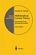Mathematical Control Theory (Eduardo D. Sontag)

This book covers what constitutes the common core of control theory and is unique in its emphasis on foundational aspects. While covering a wide range of topics written in a standard theorem/proof style, it also develops the necessary techniques from scratch.

•Feedback Control Theory (John C. Doyle, et al)

An excellent introduction to feedback control system design, this book offers a theoretical approach that captures the essential issues and can be applied to a wide range of practical problems.

•Feedback Systems: An Introduction for Scientists and Engineers

This book provides an introduction to the mathematics needed to model, analyze, and design feedback systems. It uses techniques from physics, computer science, and operations research to introduce control-oriented modeling.

•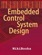Embedded Control Systems Design (Herman Bruyninckx, et al.)

The book is mainly addressed to practicing control and embedded software engineers - working in research and development – as well as graduate students who are faced with the challenge to design control systems and implement them in real-time.

•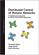Distributed Control of Robotic Networks (Francesco Bullo, et al)

This self-contained introduction to the distributed control of robotic networks offers a distinctive blend of computer science and control theory. It provides explanations of the basic concepts and main results, as well as numerous examples and exercises.

•Control Systems (WikiBooks)

This book will discuss the topic of Control Systems, which is an interdisciplinary engineering topic. Methods considered here will consist of both "Classical" control methods, and "Modern" control methods.

•Uncertainty in Engineering: Methods and Applications

This open access book provides an introduction to uncertainty quantiﬁcation in engineering. Starting with preliminaries on Bayesian statistics and Monte Carlo methods, it then focuses on reliability theory and simulation methods for complex systems.

•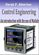Control Engineering: An Introduction with the Use of MATLAB

The book covers the basic aspects of linear single loop feedback control theory. Explanations of the mathematical concepts used in classical control such as root loci, frequency response and stability methods are explained by making use of MATLAB.

•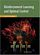Reinforcement Learning and Optimal Control (Dimitri Bertsekas)

The purpose of the book is to consider large and challenging multistage decision problems, which can be solved in principle by dynamic programming and optimal control, but their exact solution is computationally intractable.

•AI based Robot Safe Learning and Control (Xuefeng Zhou, et al)

This book mainly focuses on the safe control of robot manipulators. The control schemes are mainly developed based on dynamic neural network, which is an important theoretical branch of deep reinforcement learning.

•Discrete-Event Control of Stochastic Networks (Eitan Altman, et al)

Opening new directions in research in both discrete event dynamic systems as well as in stochastic control, this volume focuses on a wide class of control and of optimization problems over sequences of integer numbers.

•Stochastic Modeling and Control (Ivan Ganchev Ivanov)

The book provides a self-contained treatment on practical aspects of stochastic modeling and calculus including applications drawn from engineering, statistics, and computer science.

•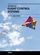Advances in Flight Control Systems (Maria Agneta Balint)

The aim of this book is to bring together reputable researchers from different countries in order to provide a comprehensive coverage of advanced and modern topics in flight control not yet reflected by other books.

•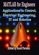MATLAB for Engineers: Applications in Control, EE, IT and Robotics

The book presents several approaches in the key areas of practice for which the MATLAB software package was used. Topics covered include applications for: Motors, Power systems , Robots, Vehicles.

•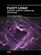Fuzzy Logic - Controls, Concepts, Theories and Applications

This book introduces new concepts and theories of Fuzzy Logic Control for the application and development of robotics and intelligent machines - how it will play a significant role in the development of intelligent robotic, machine, and mechatronics systems.

Book Categories
 :All CategoriesTop BooksRecent BooksMiscellaneous BooksComputer LanguagesComputer ScienceData Science/DatabasesElectrical EngineeringJava and Java EE (J2EE)Linux and UnixMathematicsMicrosoft and .NETMobile ComputingNetworking and CommunicationsSoftware EngineeringSpecial TopicsWeb Programming
Other Categories